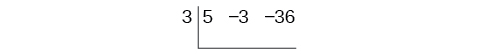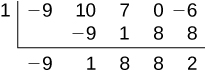5.4 Dividing polynomials  (Page 3/6)

 Page 3 / 6

Using synthetic division to divide a second-degree polynomial

Use synthetic division to divide $\text{\hspace{0.17em}}5{x}^{2}-3x-36\text{\hspace{0.17em}}$ by $\text{\hspace{0.17em}}x-3.\text{\hspace{0.17em}}$

Begin by setting up the synthetic division. Write $\text{\hspace{0.17em}}k\text{\hspace{0.17em}}$ and the coefficients.Bring down the lead coefficient. Multiply the lead coefficient by $\text{\hspace{0.17em}}k.\text{\hspace{0.17em}}$Continue by adding the numbers in the second column. Multiply the resulting number by $\text{\hspace{0.17em}}k.\text{\hspace{0.17em}}$ Write the result in the next column. Then add the numbers in the third column.The result is $\text{\hspace{0.17em}}5x+12.\text{\hspace{0.17em}}$ The remainder is 0. So $\text{\hspace{0.17em}}x-3\text{\hspace{0.17em}}$ is a factor of the original polynomial.

Using synthetic division to divide a third-degree polynomial

Use synthetic division to divide $\text{\hspace{0.17em}}4{x}^{3}+10{x}^{2}-6x-20\text{\hspace{0.17em}}$ by $\text{\hspace{0.17em}}x+2.\text{\hspace{0.17em}}$

The binomial divisor is $\text{\hspace{0.17em}}x+2\text{\hspace{0.17em}}$ so $\text{\hspace{0.17em}}k=-2.\text{\hspace{0.17em}}$ Add each column, multiply the result by –2, and repeat until the last column is reached.The result is $\text{\hspace{0.17em}}4{x}^{2}+2x-10.\text{\hspace{0.17em}}$ The remainder is 0. Thus, $\text{\hspace{0.17em}}x+2\text{\hspace{0.17em}}$ is a factor of $\text{\hspace{0.17em}}4{x}^{3}+10{x}^{2}-6x-20.\text{\hspace{0.17em}}$

Using synthetic division to divide a fourth-degree polynomial

Use synthetic division to divide $\text{\hspace{0.17em}}-9{x}^{4}+10{x}^{3}+7{x}^{2}-6\text{\hspace{0.17em}}$ by $\text{\hspace{0.17em}}x-1.\text{\hspace{0.17em}}$

Notice there is no x -term. We will use a zero as the coefficient for that term.The result is $\text{\hspace{0.17em}}-9{x}^{3}+{x}^{2}+8x+8+\frac{2}{x-1}.$

Use synthetic division to divide $\text{\hspace{0.17em}}3{x}^{4}+18{x}^{3}-3x+40\text{\hspace{0.17em}}$ by $\text{\hspace{0.17em}}x+7.$

$3{x}^{3}-3{x}^{2}+21x-150+\frac{1,090}{x+7}$

Using polynomial division to solve application problems

Polynomial division can be used to solve a variety of application problems involving expressions for area and volume. We looked at an application at the beginning of this section. Now we will solve that problem in the following example.

Using polynomial division in an application problem

The volume of a rectangular solid is given by the polynomial $\text{\hspace{0.17em}}3{x}^{4}-3{x}^{3}-33{x}^{2}+54x.\text{\hspace{0.17em}}$ The length of the solid is given by $\text{\hspace{0.17em}}3x\text{\hspace{0.17em}}$ and the width is given by $\text{\hspace{0.17em}}x-2.\text{\hspace{0.17em}}$ Find the height, $\text{\hspace{0.17em}}t,$ of the solid.

There are a few ways to approach this problem. We need to divide the expression for the volume of the solid by the expressions for the length and width. Let us create a sketch as in [link] .

We can now write an equation by substituting the known values into the formula for the volume of a rectangular solid.

$\begin{array}{ccc}\hfill V& =& l\cdot w\cdot h\hfill \\ \hfill 3{x}^{4}-3{x}^{3}-33{x}^{2}+54x& =& 3x\cdot \left(x-2\right)\cdot h\hfill \end{array}$

To solve for $\text{\hspace{0.17em}}h,\text{\hspace{0.17em}}$ first divide both sides by $\text{\hspace{0.17em}}3x.$

$\begin{array}{ccc}\hfill \frac{3x\cdot \left(x-2\right)\cdot h}{3x}& =& \frac{3{x}^{4}-3{x}^{3}-33{x}^{2}+54x}{3x}\hfill \\ \hfill \left(x-2\right)h& =& {x}^{3}-{x}^{2}-11x+18\hfill \end{array}$

Now solve for $\text{\hspace{0.17em}}h\text{\hspace{0.17em}}$ using synthetic division.

$h=\frac{{x}^{3}-{x}^{2}-11x+18}{x-2}$The quotient is $\text{\hspace{0.17em}}{x}^{2}+x-9\text{\hspace{0.17em}}$ and the remainder is 0. The height of the solid is $\text{\hspace{0.17em}}{x}^{2}+x-9.$

The area of a rectangle is given by $\text{\hspace{0.17em}}3{x}^{3}+14{x}^{2}-23x+6.\text{\hspace{0.17em}}$ The width of the rectangle is given by $\text{\hspace{0.17em}}x+6.\text{\hspace{0.17em}}$ Find an expression for the length of the rectangle.

$3{x}^{2}-4x+1$

Access these online resources for additional instruction and practice with polynomial division.

Key equations

 Division Algorithm

Key concepts

• Polynomial long division can be used to divide a polynomial by any polynomial with equal or lower degree. See [link] and [link] .
• The Division Algorithm tells us that a polynomial dividend can be written as the product of the divisor and the quotient added to the remainder.
• Synthetic division is a shortcut that can be used to divide a polynomial by a binomial in the form $\text{\hspace{0.17em}}x-k.\text{\hspace{0.17em}}$ See [link] , [link] , and [link] .
• Polynomial division can be used to solve application problems, including area and volume. See [link] .

A laser rangefinder is locked on a comet approaching Earth. The distance g(x), in kilometers, of the comet after x days, for x in the interval 0 to 30 days, is given by g(x)=250,000csc(π30x). Graph g(x) on the interval [0, 35]. Evaluate g(5)  and interpret the information. What is the minimum distance between the comet and Earth? When does this occur? To which constant in the equation does this correspond? Find and discuss the meaning of any vertical asymptotes.
The sequence is {1,-1,1-1.....} has
how can we solve this problem
Sin(A+B) = sinBcosA+cosBsinA
Prove it
Eseka
Eseka
hi
Joel
June needs 45 gallons of punch. 2 different coolers. Bigger cooler is 5 times as large as smaller cooler. How many gallons in each cooler?
7.5 and 37.5
Nando
find the sum of 28th term of the AP 3+10+17+---------
I think you should say "28 terms" instead of "28th term"
Vedant
the 28th term is 175
Nando
192
Kenneth
if sequence sn is a such that sn>0 for all n and lim sn=0than prove that lim (s1 s2............ sn) ke hole power n =n
write down the polynomial function with root 1/3,2,-3 with solution
if A and B are subspaces of V prove that (A+B)/B=A/(A-B)
write down the value of each of the following in surd form a)cos(-65°) b)sin(-180°)c)tan(225°)d)tan(135°)
Prove that (sinA/1-cosA - 1-cosA/sinA) (cosA/1-sinA - 1-sinA/cosA) = 4
what is the answer to dividing negative index
In a triangle ABC prove that. (b+c)cosA+(c+a)cosB+(a+b)cisC=a+b+c.
give me the waec 2019 questionsByByByBy Edward BitonByByBy Rhodes